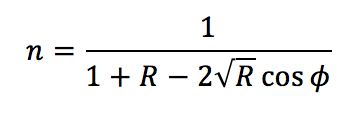# Estimation of the Refractive Index of a Monocrystalline Sapphire via Polarization Measurement using the MSV-5000 Series Microscopic Spectrophotometer

August 18, 2022

## Introduction

The MSV-5000 series microscopic spectrophotometer is for transmission and reflection measurements in a wide wavelength range from the ultraviolet to near infrared. The high resolution camera enables sample areas as small as 10  μm in diameter. The built-in Glan-Taylor polarizer allows optical constants such as refractive index and extinction coefficient to be obtained by measuring the reflectance spectrum of birefringent samples.

In this application note, a 50 μm monocrystalline sapphire, which has two types of crystal axes, was measured and the dispersion of the refractive index was calculated.

## Experimental

Two baselines of an aluminum vapor-deposited mirror was measured with the polarizer angle at 0 and 90º as a reference. To determine the crystalline axis, the polarizer angle was set to 0º and the wavelength 550 nm. The sample was then rotated to find the angle where the sample displayed maximum reflectance. The c-axis was determined by this angle and the a-axis was taken orthogonal to it. The sample reflectance spectra were obtained with polarizer angles at 0 and 90º for the c- and a- axis, respectively. The sample absolute reflectance spectrum was calculated by multiplying the obtained relative reflectance by the absolute reflectance spectrum of the aluminum vapor-deposited mirror.

The refractive index can be calculated by either the UV-Vis K-K Conversion program or Multi-Layer Analysis program. Using the UV-Vis K-K Conversion program, the refractive index (n) is expressed by the specular reflectance spectrum (R) and phase change (Φ):The phase change was calculated using the Kramers-Kronig conversion of the specular reflectance spectrum:and then the refractive index (n) was calculated.

Using the Multi-Layer Analysis program, the reflectance spectrum is expressed by the refractive index of air and the sample (n1, n2), the indicence angle (θ1), and the reflection angle (θ2):By applying this equation, the wavelength dispersion of the refractive index is calculated using the Multi-Layer Analysis program by fitting the calculated reflectance spectrum to the measured.

## Keywords

UV-0025, MSV-5200 UV-Vis/NIR microscopic spectrophotometer, VWML-791 Multi-layer Analysis program, Materials

## Results

The absolute reflectance spectrum of monocrystalline sapphire is shown in Figure 1. The reflectance of the ordinary light (c-axis) is approximately 0.15% higher than that of the extraordinary light (a-axis).

Using the UV-Vis K-K Conversion and Multi-Layer Analysis program, the wavelength dispersion of the refractive index was obtained and shown in Figure 2. Tables 1 and 2 illustrate the results compared with literature values of the refractive index of ordinary and extraordinary light. The refractive index was determined within two decimal places in an area several tens of microns in diameter.Figure 2. Wavelength dispersion of the refractive index calculated using the UV-Vis K-K Conversion program (left) and Multi-Layer Analysis program (right).

Table 1. Comparison of the calculated refractive index values of ordinary light (c-axis) using the UV-Vis K-K Conversion program with the literature values.

 Wavelength Literature Value K-K Transform Multilayer Analysis Refractive Index Refractive Index Error Refractive Index Error 632.8 1.776 1.770 0.0037 1.769 0.0036 589.3 1.768 1.770 0.0016 1.771 0.0028 546.1 1.771 1.772 0.0012 1.773 0.0018 532.0 1,771 1.771 -0.0005 1.773 0.0015 514.5 1.773 1.773 -0.0005 1.774 0.0011 488.0 1.775 1.775 -0.0005 1.776 0.0007

Table 2. Comparison of the calculated refractive index values of unordinary light (c-axis) using the UV-Vis K-K Conversion program with the literature values.

 Wavelength Literature Value K-K Transform Multilayer Analysis Refractive Index Refractive Index Error Refractive Index Error 632.8 1.758 1.757 -0.0005 1.758 -0.0003 589.3 1.760 1.758 -0.0015 1.759 -0.0011 546.1 1.763 1.761 -0.0017 1.761 -0.0020 532.0 1,764 1.761 -0.0030 1.761 -0.0022 514.5 1.765 1.762 -0.0032 1.762 -0.0026 488.0 1.767 1.764 -0.0028 1.764 -0.0030